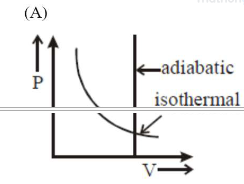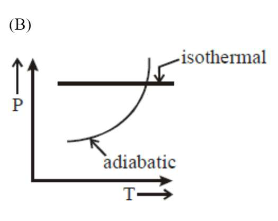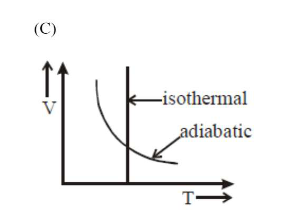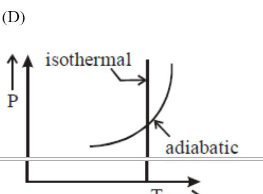# Which one is the correct option for the two different thermodynamic processes?Question:

Which one is the correct option for the two different thermodynamic processes?1. (C) and (A)

2. (C) and (D)

3. (A) only

4. $(B)$ and $(C)$

Correct Option: , 2

Solution:

(2)

Option (a) is wrong; since in adiabatic

process $V \neq$ constant.

Option (b) is wrong, since in isothermal process

$T=$ constant

Option (c) $\backslash \&$

(d) matches isothermes $\backslash \&$

constant $\backslash \& \frac{\mathrm{T}^{\gamma}}{\mathrm{p}^{\gamma-1}}=$ constant